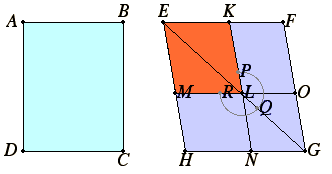# Definition 1

Any rectangular parallelogram is said to be contained by the two straight lines containing the right angle.

# Definition 2

And in any parallelogrammic area let any one whatever of the parallelograms about its diameter with the two complements be called a gnomon.

### Guide

Euclid didn’t a single word for rectangle, but two words meaning right-angled parallelogram.According to the first definition, the rectangle ABCD illustrated on the left is contained by the lines AB and BC, and this rectangle can be called the rectangle AB by BC. Of course, it could also be called the rectangle BC by CD, or two other names.

On the right, in the parallelogram EFGH, there is a diameter EG with a parallelogram LNGO about it and the two complements KLOF and MHNL, and these three parallelograms together make up the gnomon. In other words a gnomon is an L-shaped figure made by removing a parallelogram from a larger similar parallelogram.

The word gnomon comes from the Greek word meaning carpenter’s square. A carpenter’s square is an L-shaped tool with its two sides at right angles. Euclid’s use of the word is looser as he doesn’t require that the sides be at right angles.

Euclid illustrated gnomons by arcs of circles around the inner vertex. In this example, the gnomon is called the gnomon PQR.